# RedisConf18 - Lower Latency Graph Queries in Cypher with Redis Graph

May. 30, 2018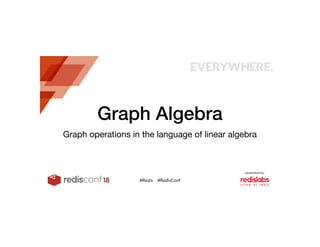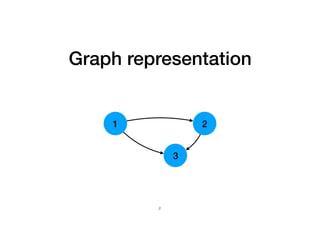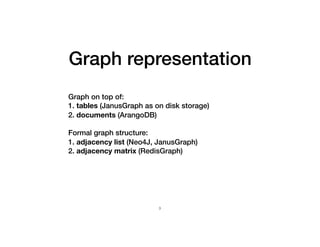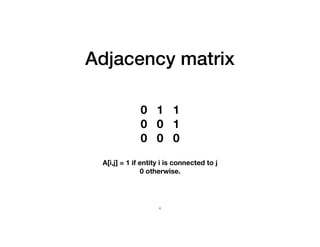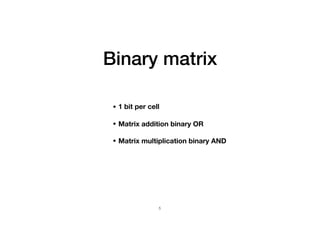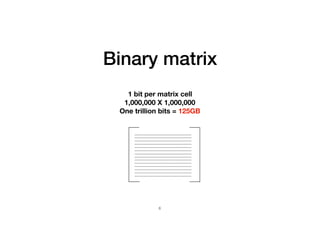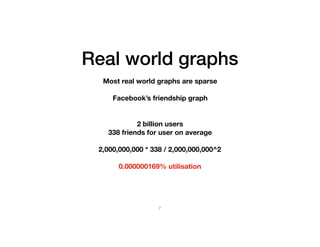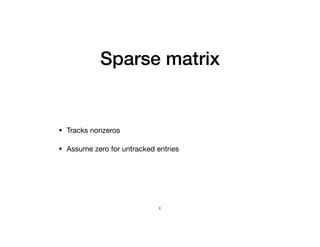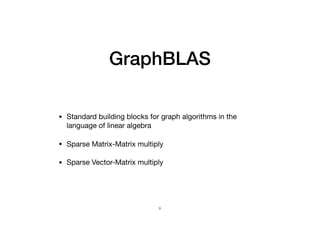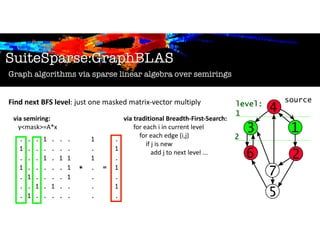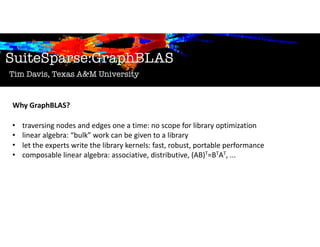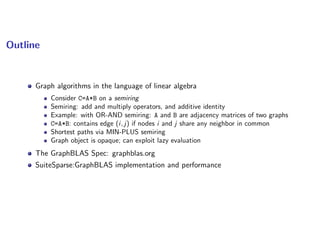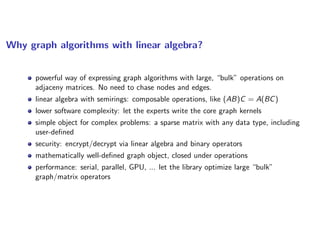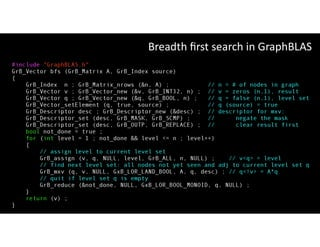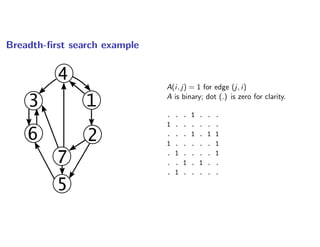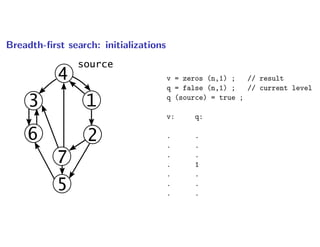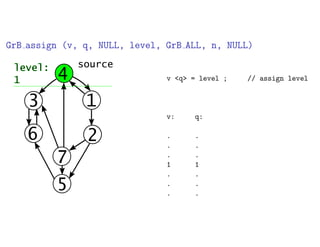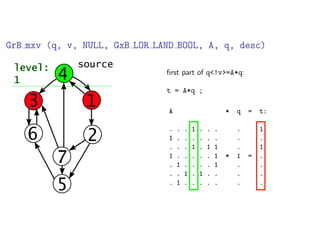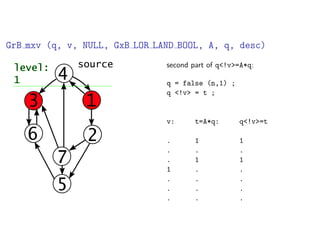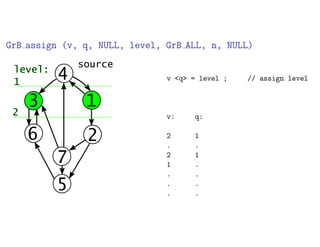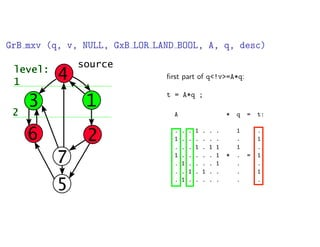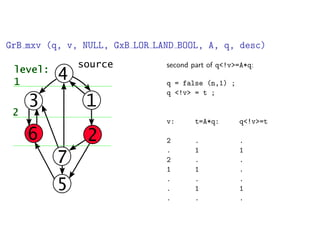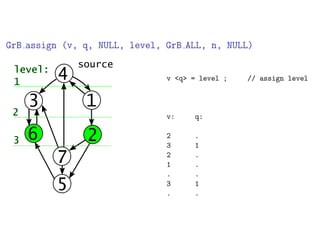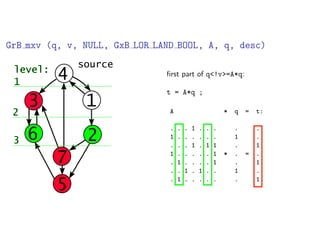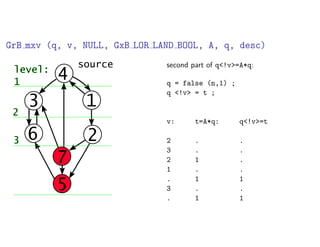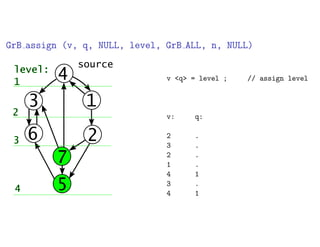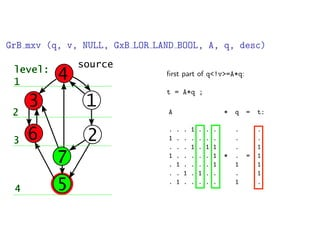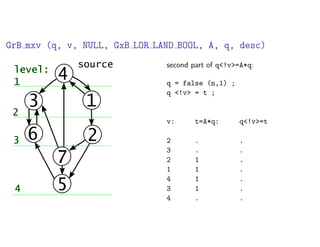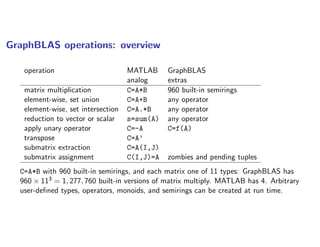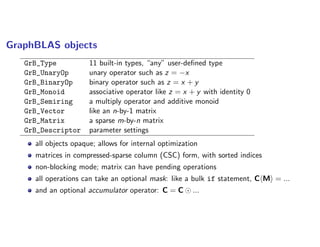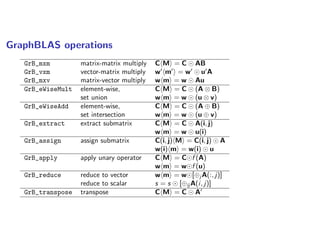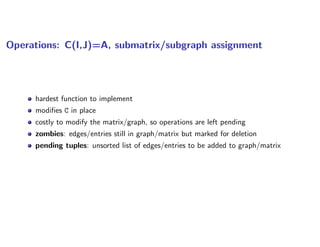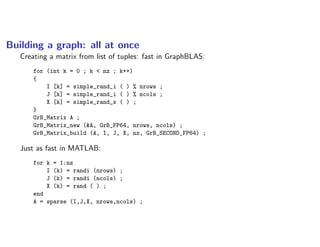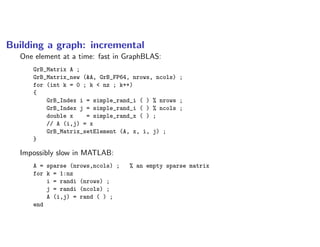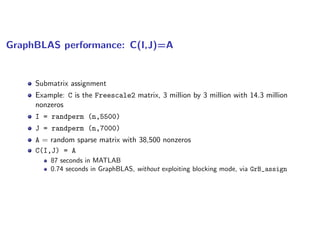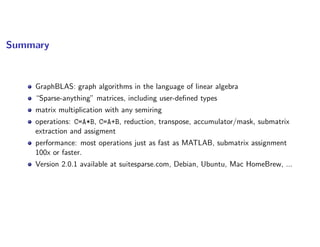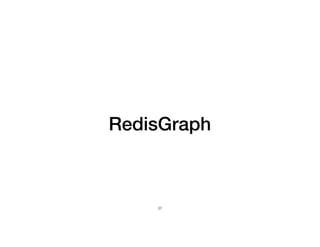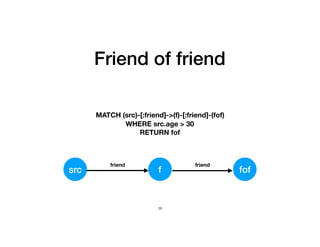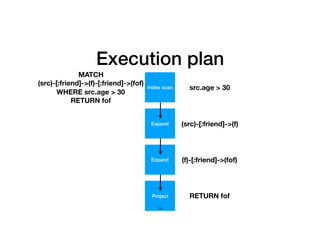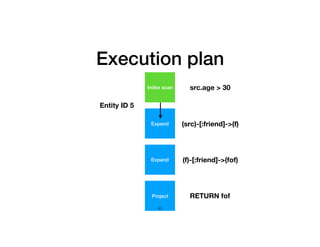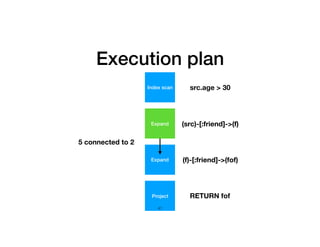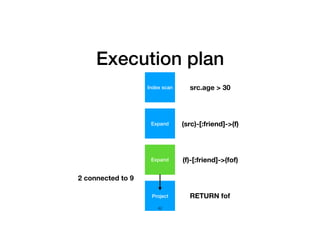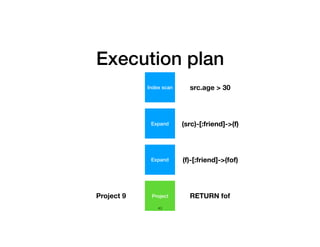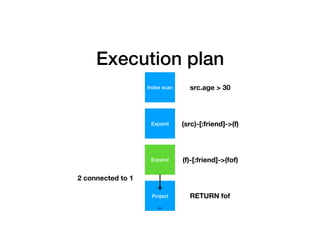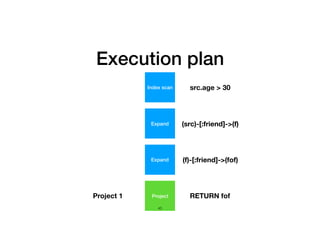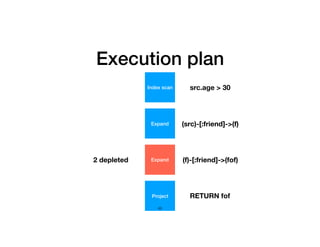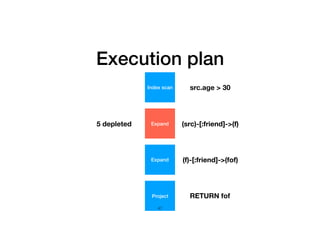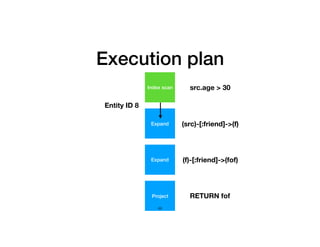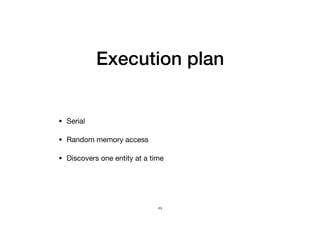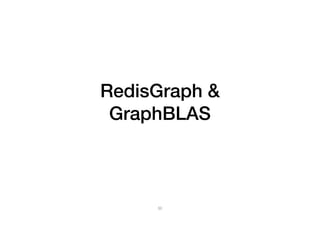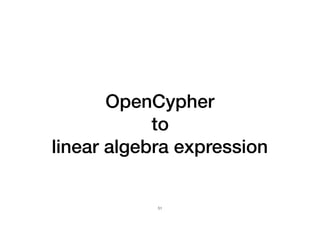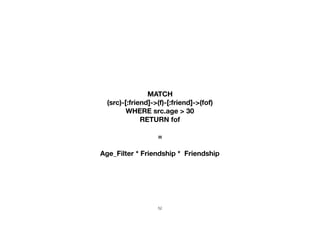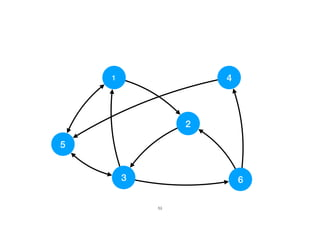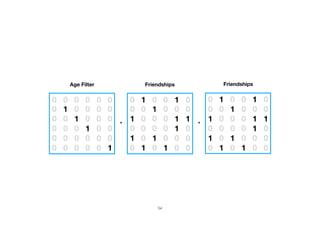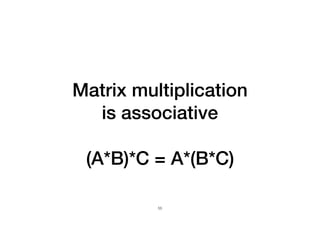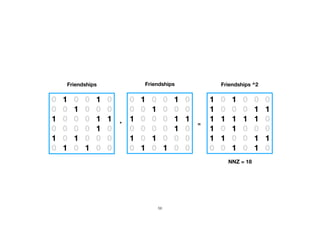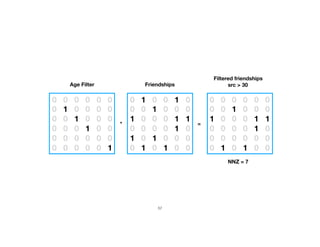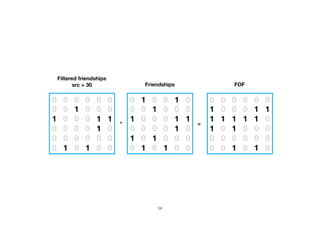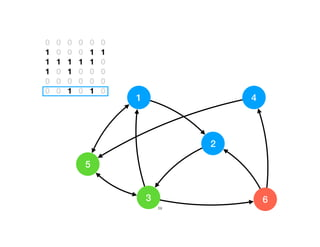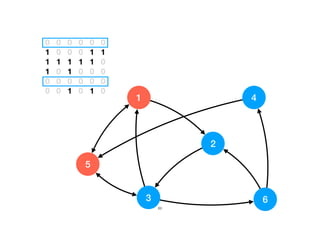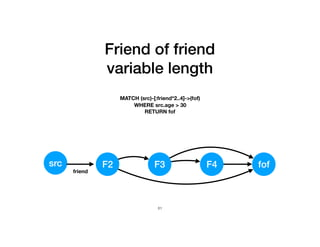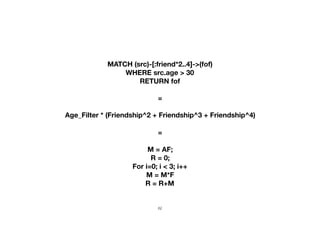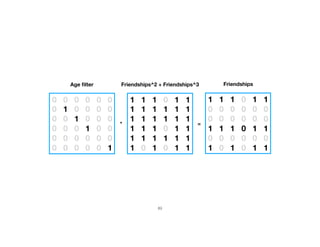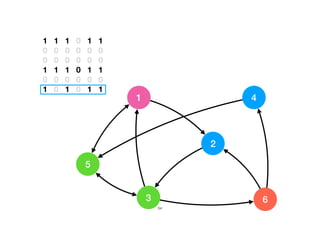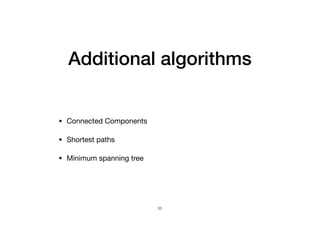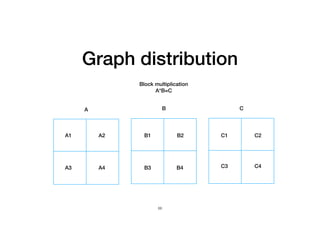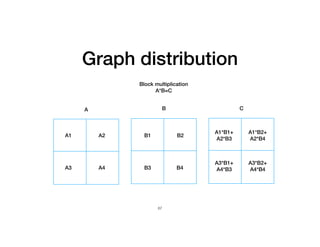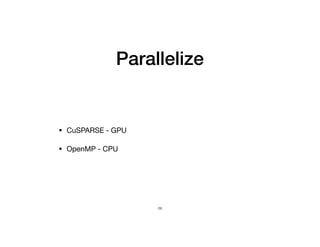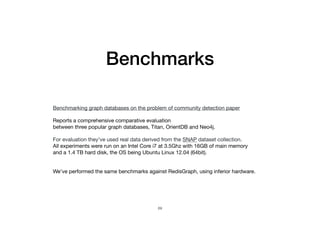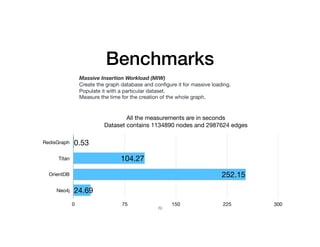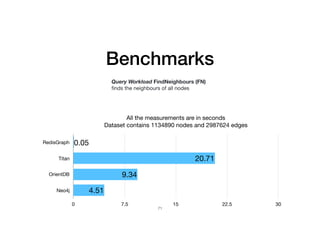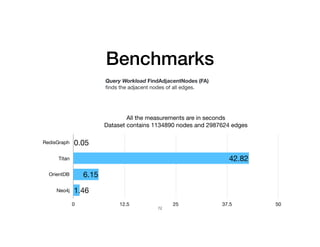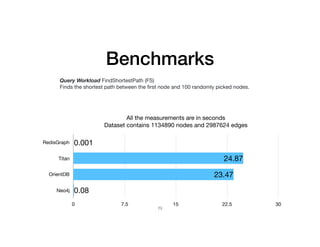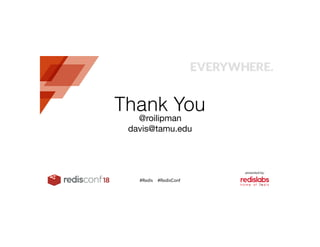1 of 74

### RedisConf18 - Lower Latency Graph Queries in Cypher with Redis Graph

1. Graph Algebra Graph operations in the language of linear algebra 1
2. Graph representation 1 2 3 2
3. Graph representation Graph on top of:  1. tables (JanusGraph as on disk storage) 2. documents (ArangoDB) Formal graph structure: 1. adjacency list (Neo4J, JanusGraph) 2. adjacency matrix (RedisGraph) 3
4. Adjacency matrix 0 1 1 0 0 1 0 0 0 A[i,j] = 1 if entity i is connected to j 0 otherwise. 4
5. Binary matrix • 1 bit per cell • Matrix addition binary OR  • Matrix multiplication binary AND 5
6. Binary matrix 1 bit per matrix cell 1,000,000 X 1,000,000 One trillion bits = 125GB ………………………………………………………. ………………………………………………………. ………………………………………………………. ………………………………………………………. ………………………………………………………. ………………………………………………………. ………………………………………………………. ………………………………………………………. ………………………………………………………. ………………………………………………………. ………………………………………………………. ………………………………………………………. ………………………………………………………. ………………………………………………………. 6
7. Real world graphs Most real world graphs are sparse Facebook’s friendship graph 2 billion users 338 friends for user on average 2,000,000,000 * 338 / 2,000,000,000^2 0.000000169% utilisation 7
8. Sparse matrix • Tracks nonzeros • Assume zero for untracked entries 8
9. GraphBLAS • Standard building blocks for graph algorithms in the language of linear algebra • Sparse Matrix-Matrix multiply • Sparse Vector-Matrix multiply 9
10. SuiteSparse:GraphBLAS Graph algorithms via sparse linear algebra over semirings via traditional Breadth-First-Search: for each i in current level for each edge (i,j) if j is new add j to next level ... Find next BFS level: just one masked matrix-vector multiply Tim Davis, Texas A&M University via semiring: y<mask>=A*x
11. SuiteSparse:GraphBLAS • traversing nodes and edges one a time: no scope for library optimization • linear algebra: “bulk” work can be given to a library • let the experts write the library kernels: fast, robust, portable performance • composable linear algebra: associative, distributive, (AB)T=BTAT, ... Tim Davis, Texas A&M University Why GraphBLAS?
12. Outline Graph algorithms in the language of linear algebra Consider C=A*B on a semiring Semiring: add and multiply operators, and additive identity Example: with OR-AND semiring: A and B are adjacency matrices of two graphs C=A*B: contains edge (i, j) if nodes i and j share any neighbor in common Shortest paths via MIN-PLUS semiring Graph object is opaque; can exploit lazy evaluation The GraphBLAS Spec: graphblas.org SuiteSparse:GraphBLAS implementation and performance
13. Why graph algorithms with linear algebra? powerful way of expressing graph algorithms with large, “bulk” operations on adjaceny matrices. No need to chase nodes and edges. linear algebra with semirings: composable operations, like (AB)C = A(BC) lower software complexity: let the experts write the core graph kernels simple object for complex problems: a sparse matrix with any data type, including user-deﬁned security: encrypt/decrypt via linear algebra and binary operators mathematically well-deﬁned graph object, closed under operations performance: serial, parallel, GPU, ... let the library optimize large “bulk” graph/matrix operators
14. Breadth-ﬁrst search example A(i, j) = 1 for edge (j, i) A is binary; dot (.) is zero for clarity. . . . 1 . . . 1 . . . . . . . . . 1 . 1 1 1 . . . . . 1 . 1 . . . . 1 . . 1 . 1 . . . 1 . . . . .
15. Breadth-ﬁrst search: initializations v = zeros (n,1) ; // result q = false (n,1) ; // current level q (source) = true ; v: q: . . . . . . . 1 . . . . . .
16. GrB assign (v, q, NULL, level, GrB ALL, n, NULL) v <q> = level ; // assign level v: q: . . . . . . 1 1 . . . . . .
17. GrB mxv (q, v, NULL, GxB LOR LAND BOOL, A, q, desc) ﬁrst part of q<!v>=A*q: t = A*q ;
18. GrB mxv (q, v, NULL, GxB LOR LAND BOOL, A, q, desc) second part of q<!v>=A*q: q = false (n,1) ; q <!v> = t ; v: t=A*q: q<!v>=t . 1 1 . . . . 1 1 1 . . . . . . . . . . .
19. GrB assign (v, q, NULL, level, GrB ALL, n, NULL) v <q> = level ; // assign level v: q: 2 1 . . 2 1 1 . . . . . . .
20. GrB mxv (q, v, NULL, GxB LOR LAND BOOL, A, q, desc) ﬁrst part of q<!v>=A*q: t = A*q ;
21. GrB mxv (q, v, NULL, GxB LOR LAND BOOL, A, q, desc) second part of q<!v>=A*q: q = false (n,1) ; q <!v> = t ; v: t=A*q: q<!v>=t 2 . . . 1 1 2 . . 1 1 . . . . . 1 1 . . .
22. GrB assign (v, q, NULL, level, GrB ALL, n, NULL) v <q> = level ; // assign level v: q: 2 . 3 1 2 . 1 . . . 3 1 . .
23. GrB mxv (q, v, NULL, GxB LOR LAND BOOL, A, q, desc) ﬁrst part of q<!v>=A*q: t = A*q ;
24. GrB mxv (q, v, NULL, GxB LOR LAND BOOL, A, q, desc) second part of q<!v>=A*q: q = false (n,1) ; q <!v> = t ; v: t=A*q: q<!v>=t 2 . . 3 . . 2 1 . 1 . . . 1 1 3 . . . 1 1
25. GrB assign (v, q, NULL, level, GrB ALL, n, NULL) v <q> = level ; // assign level v: q: 2 . 3 . 2 . 1 . 4 1 3 . 4 1
26. GrB mxv (q, v, NULL, GxB LOR LAND BOOL, A, q, desc) ﬁrst part of q<!v>=A*q: t = A*q ;
27. GrB mxv (q, v, NULL, GxB LOR LAND BOOL, A, q, desc) second part of q<!v>=A*q: q = false (n,1) ; q <!v> = t ; v: t=A*q: q<!v>=t 2 . . 3 . . 2 1 . 1 1 . 4 1 . 3 1 . 4 . .
28. GraphBLAS operations: overview operation MATLAB GraphBLAS analog extras matrix multiplication C=A*B 960 built-in semirings element-wise, set union C=A+B any operator element-wise, set intersection C=A.*B any operator reduction to vector or scalar s=sum(A) any operator apply unary operator C=-A C=f(A) transpose C=A’ submatrix extraction C=A(I,J) submatrix assignment C(I,J)=A zombies and pending tuples C=A*B with 960 built-in semirings, and each matrix one of 11 types: GraphBLAS has 960 ⇥ 113 = 1, 277, 760 built-in versions of matrix multiply. MATLAB has 4. Arbitrary user-deﬁned types, operators, monoids, and semirings can be created at run time.
29. GraphBLAS objects GrB_Type 11 built-in types, “any” user-deﬁned type GrB_UnaryOp unary operator such as z = x GrB_BinaryOp binary operator such as z = x + y GrB_Monoid associative operator like z = x + y with identity 0 GrB_Semiring a multiply operator and additive monoid GrB_Vector like an n-by-1 matrix GrB_Matrix a sparse m-by-n matrix GrB_Descriptor parameter settings all objects opaque; allows for internal optimization matrices in compressed-sparse column (CSC) form, with sorted indices non-blocking mode; matrix can have pending operations all operations can take an optional mask: like a bulk if statement, ChMi = ... and an optional accumulator operator: C = C ...
30. GraphBLAS operations GrB_mxm matrix-matrix multiply ChMi = C AB GrB_vxm vector-matrix multiply w0 hm0 i = w0 u0 A GrB_mxv matrix-vector multiply whmi = w Au GrB_eWiseMult element-wise, ChMi = C (A ⌦ B) set union whmi = w (u ⌦ v) GrB_eWiseAdd element-wise, ChMi = C (A B) set intersection whmi = w (u v) GrB_extract extract submatrix ChMi = C A(i, j) whmi = w u(i) GrB_assign assign submatrix C(i, j)hMi = C(i, j) A w(i)hmi = w(i) u GrB_apply apply unary operator ChMi = C f (A) whmi = w f (u) GrB_reduce reduce to vector whmi = w [ j A(:, j)] reduce to scalar s = s [ ij A(i, j)] GrB_transpose transpose ChMi = C A0
31. Operations: C(I,J)=A, submatrix/subgraph assignment hardest function to implement modiﬁes C in place costly to modify the matrix/graph, so operations are left pending zombies: edges/entries still in graph/matrix but marked for deletion pending tuples: unsorted list of edges/entries to be added to graph/matrix
32. Building a graph: all at once Creating a matrix from list of tuples: fast in GraphBLAS: for (int k = 0 ; k < nz ; k++) { I [k] = simple_rand_i ( ) % nrows ; J [k] = simple_rand_i ( ) % ncols ; X [k] = simple_rand_x ( ) ; } GrB_Matrix A ; GrB_Matrix_new (&A, GrB_FP64, nrows, ncols) ; GrB_Matrix_build (A, I, J, X, nz, GrB_SECOND_FP64) ; Just as fast in MATLAB: for k = 1:nz I (k) = randi (nrows) ; J (k) = randi (ncols) ; X (k) = rand ( ) ; end A = sparse (I,J,X, nrows,ncols) ;
33. Building a graph: incremental One element at a time: fast in GraphBLAS: GrB_Matrix A ; GrB_Matrix_new (&A, GrB_FP64, nrows, ncols) ; for (int k = 0 ; k < nz ; k++) { GrB_Index i = simple_rand_i ( ) % nrows ; GrB_Index j = simple_rand_i ( ) % ncols ; double x = simple_rand_x ( ) ; // A (i,j) = x GrB_Matrix_setElement (A, x, i, j) ; } Impossibly slow in MATLAB: A = sparse (nrows,ncols) ; % an empty sparse matrix for k = 1:nz i = randi (nrows) ; j = randi (ncols) ; A (i,j) = rand ( ) ; end
34. GraphBLAS performance: C(I,J)=A Submatrix assignment Example: C is the Freescale2 matrix, 3 million by 3 million with 14.3 million nonzeros I = randperm (n,5500) J = randperm (n,7000) A = random sparse matrix with 38,500 nonzeros C(I,J) = A 87 seconds in MATLAB 0.74 seconds in GraphBLAS, without exploiting blocking mode, via GrB_assign
35. Summary GraphBLAS: graph algorithms in the language of linear algebra “Sparse-anything” matrices, including user-deﬁned types matrix multiplication with any semiring operations: C=A*B, C=A+B, reduction, transpose, accumulator/mask, submatrix extraction and assigment performance: most operations just as fast as MATLAB, submatrix assignment 100x or faster. Version 2.0.1 available at suitesparse.com, Debian, Ubuntu, Mac HomeBrew, ...
36. RedisGraph 37
37. Friend of friend MATCH (src)-[:friend]->(f)-[:friend]-(fof) WHERE src.age > 30 RETURN fof src f fof friend friend 38
38. Execution plan MATCH  (src)-[:friend]->(f)-[:friend]->(fof) WHERE src.age > 30 RETURN fof Index scan Expand Expand Project src.age > 30 (src)-[:friend]->(f) (f)-[:friend]->(fof) RETURN fof 39
39. Execution plan Index scan Expand Expand Project Entity ID 5 40 src.age > 30 (src)-[:friend]->(f) (f)-[:friend]->(fof) RETURN fof
40. Execution plan Index scan Expand Expand Project 5 connected to 2 41 src.age > 30 (src)-[:friend]->(f) (f)-[:friend]->(fof) RETURN fof
41. Execution plan Index scan Expand Expand Project 2 connected to 9 42 src.age > 30 (src)-[:friend]->(f) (f)-[:friend]->(fof) RETURN fof
42. Execution plan Index scan Expand Expand ProjectProject 9 43 src.age > 30 (src)-[:friend]->(f) (f)-[:friend]->(fof) RETURN fof
43. Execution plan Index scan Expand Expand Project 2 connected to 1 44 src.age > 30 (src)-[:friend]->(f) (f)-[:friend]->(fof) RETURN fof
44. Execution plan Index scan Expand Expand ProjectProject 1 45 src.age > 30 (src)-[:friend]->(f) (f)-[:friend]->(fof) RETURN fof
45. Execution plan Index scan Expand Expand Project 2 depleted 46 src.age > 30 (src)-[:friend]->(f) (f)-[:friend]->(fof) RETURN fof
46. Execution plan Index scan Expand Expand Project 5 depleted 47 src.age > 30 (src)-[:friend]->(f) (f)-[:friend]->(fof) RETURN fof
47. Execution plan Index scan Expand Expand Project Entity ID 8 48 src.age > 30 (src)-[:friend]->(f) (f)-[:friend]->(fof) RETURN fof
48. Execution plan • Serial • Random memory access • Discovers one entity at a time 49
49. RedisGraph & GraphBLAS 50
50. OpenCypher to  linear algebra expression 51
51. MATCH  (src)-[:friend]->(f)-[:friend]->(fof) WHERE src.age > 30 RETURN fof = Age_Filter * Friendship * Friendship 52
52. 1 5 4 2 63 53
53. 0 0 0 0 0 0 0 1 0 0 0 0 0 0 1 0 0 0 0 0 0 1 0 0 0 0 0 0 0 0 0 0 0 0 0 1 Age Filter 0 1 0 0 1 0 0 0 1 0 0 0 1 0 0 0 1 1 0 0 0 0 1 0 1 0 1 0 0 0 0 1 0 1 0 0 Friendships 0 1 0 0 1 0 0 0 1 0 0 0 1 0 0 0 1 1 0 0 0 0 1 0 1 0 1 0 0 0 0 1 0 1 0 0 Friendships * * 54
54. Matrix multiplication is associative (A*B)*C = A*(B*C) 55
55. 0 1 0 0 1 0 0 0 1 0 0 0 1 0 0 0 1 1 0 0 0 0 1 0 1 0 1 0 0 0 0 1 0 1 0 0 Friendships 0 1 0 0 1 0 0 0 1 0 0 0 1 0 0 0 1 1 0 0 0 0 1 0 1 0 1 0 0 0 0 1 0 1 0 0 Friendships * 1 0 1 0 0 0 1 0 0 0 1 1 1 1 1 1 1 0 1 0 1 0 0 0 1 1 0 0 1 1 0 0 1 0 1 0 Friendships ^2 = NNZ = 18 56
56. Age Filter 0 1 0 0 1 0 0 0 1 0 0 0 1 0 0 0 1 1 0 0 0 0 1 0 1 0 1 0 0 0 0 1 0 1 0 0 Friendships 0 0 0 0 0 0 0 0 1 0 0 0 1 0 0 0 1 1 0 0 0 0 1 0 0 0 0 0 0 0 0 1 0 1 0 0 Filtered friendships  src > 30 * = NNZ = 7 0 0 0 0 0 0 0 1 0 0 0 0 0 0 1 0 0 0 0 0 0 1 0 0 0 0 0 0 0 0 0 0 0 0 0 1 57
57. 0 1 0 0 1 0 0 0 1 0 0 0 1 0 0 0 1 1 0 0 0 0 1 0 1 0 1 0 0 0 0 1 0 1 0 0 Friendships 0 0 0 0 0 0 0 0 1 0 0 0 1 0 0 0 1 1 0 0 0 0 1 0 0 0 0 0 0 0 0 1 0 1 0 0 Filtered friendships  src > 30 * = 0 0 0 0 0 0 1 0 0 0 1 1 1 1 1 1 1 0 1 0 1 0 0 0 0 0 0 0 0 0 0 0 1 0 1 0 FOF 58
58. 0 0 0 0 0 0 1 0 0 0 1 1 1 1 1 1 1 0 1 0 1 0 0 0 0 0 0 0 0 0 0 0 1 0 1 0 1 5 4 2 63 59
59. 0 0 0 0 0 0 1 0 0 0 1 1 1 1 1 1 1 0 1 0 1 0 0 0 0 0 0 0 0 0 0 0 1 0 1 0 1 5 4 2 63 60
60. Friend of friend variable length MATCH (src)-[:friend*2..4]->(fof) WHERE src.age > 30 RETURN fof src F2 fof friend F3 F4 61
61. MATCH (src)-[:friend*2..4]->(fof) WHERE src.age > 30 RETURN fof = Age_Filter * (Friendship^2 + Friendship^3 + Friendship^4)   = M = AF;  R = 0;  For i=0; i < 3; i++  M = M*F  R = R+M 62
62. 0 0 0 0 0 0 0 1 0 0 0 0 0 0 1 0 0 0 0 0 0 1 0 0 0 0 0 0 0 0 0 0 0 0 0 1 Age ﬁlter 1 1 1 0 1 1 1 1 1 1 1 1 1 1 1 1 1 1 1 1 1 0 1 1 1 1 1 1 1 1 1 0 1 0 1 1 Friendships^2 + Friendships^3 1 1 1 0 1 1 0 0 0 0 0 0 0 0 0 0 0 0 1 1 1 0 1 1 0 0 0 0 0 0 1 0 1 0 1 1 Friendships * = 63
63. 1 5 4 2 63 1 1 1 0 1 1 0 0 0 0 0 0 0 0 0 0 0 0 1 1 1 0 1 1 0 0 0 0 0 0 1 0 1 0 1 1 64
64. Additional algorithms • Connected Components • Shortest paths • Minimum spanning tree 65
65. Graph distribution Block multiplication A*B=C A B C A1 A3 A4 A2 B1 B2 B4B3 C1 C2 C3 C4 66
66. Graph distribution Block multiplication A*B=C A B C A1 A3 A4 A2 B1 B2 B4B3 A1*B1+  A2*B3 A1*B2+  A2*B4 A3*B1+  A4*B3 A3*B2+  A4*B4 67
67. Parallelize • CuSPARSE - GPU • OpenMP - CPU 68
68. Benchmarks 69 Benchmarking graph databases on the problem of community detection paper Reports a comprehensive comparative evaluation  between three popular graph databases, Titan, OrientDB and Neo4j. For evaluation they’ve used real data derived from the SNAP dataset collection. All experiments were run on an Intel Core i7 at 3.5Ghz with 16GB of main memory  and a 1.4 TB hard disk, the OS being Ubuntu Linux 12.04 (64bit). We’ve performed the same benchmarks against RedisGraph, using inferior hardware.
69. Benchmarks 70 Massive Insertion Workload (MIW) Create the graph database and conﬁgure it for massive loading. Populate it with a particular dataset. Measure the time for the creation of the whole graph. All the measurements are in seconds  Dataset contains 1134890 nodes and 2987624 edges RedisGraph Titan OrientDB Neo4j 0 75 150 225 300 24.69 252.15 104.27 0.53
70. Benchmarks 71 Query Workload FindNeighbours (FN)  ﬁnds the neighbours of all nodes All the measurements are in seconds  Dataset contains 1134890 nodes and 2987624 edges RedisGraph Titan OrientDB Neo4j 0 7.5 15 22.5 30 4.51 9.34 20.71 0.05
71. Benchmarks 72 Query Workload FindAdjacentNodes (FA)  ﬁnds the adjacent nodes of all edges. All the measurements are in seconds  Dataset contains 1134890 nodes and 2987624 edges RedisGraph Titan OrientDB Neo4j 0 12.5 25 37.5 50 1.46 6.15 42.82 0.05
72. Benchmarks 73 Query Workload FindShortestPath (FS)  Finds the shortest path between the ﬁrst node and 100 randomly picked nodes. All the measurements are in seconds  Dataset contains 1134890 nodes and 2987624 edges RedisGraph Titan OrientDB Neo4j 0 7.5 15 22.5 30 0.08 23.47 24.87 0.001
73. Thank You @roilipman davis@tamu.edu 74